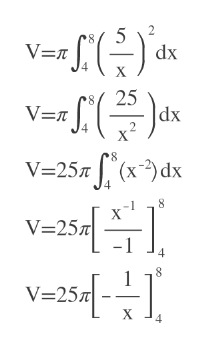# Consider the solid obtained by rotating the region bounded by the given curves about the x-axis.y = 5/x, x = 4, x = 8, y = 0 Find the volume V of this solid.

Question
1 views

Consider the solid obtained by rotating the region bounded by the given curves about the x-axis.
y = 5/x, x = 4, x = 8, y = 0
Find the volume V of this solid.

check_circle

Step 1

We will have to use Disk method with a=4, b=8

y=5/x given

Step 2

Then we calculate t...help_outlineImage Transcriptionclose2 5 dx V=R X 25 |dx V=n 2 8 V=257(x)dx X V-25 8 V 25 X fullscreen

### Want to see the full answer?

See Solution

#### Want to see this answer and more?

Solutions are written by subject experts who are available 24/7. Questions are typically answered within 1 hour.*

See Solution
*Response times may vary by subject and question.
Tagged in

### Other